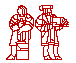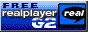#Professor Strang's Linear Algebra Class Lecture Videos

Welcome to the Videotaped Lectures webpage for MIT's Course 18.06: Linear Algebra .

Standard Athena workstation configurations will allow you to view the 18.06 lecture videos. If you wish to access the videos from a Mac or PC, you should download the RealPlayer. You do not need the Plus Version of the RealPlayer - the free RealPlayer will work fine.Lecture #1: The Geometry of Linear Equations (56k)|(80k)|(220k) Lecture #19: Determinant Formulas and Cofactors (56k)|(80k)|(220k) Lecture #2: Elimination with Matrices (56k)|(80k)|(220k) Lecture #20: Cramer's Rule, Inverse Matrix, and Volume (56k)|(80k)|(220k) Lecture #3: Multiplication and Inverse Matrices (56k)|(80k)|(220k) Lecture #21: Eigenvalues and Eigenvectors (56k)|(80k)|(220k) Lecture #4: Factorization into A = LU (56k)|(80k)|(220k) Lecture #22: Diagonalization and Powers of A (56k)|(80k)|(220k) Lecture #5: Transposes, Permutations, Spaces R^n (56k)|(80k)|(220k) Lecture #23: Differential Equations and exp(At) (56k)|(80k)|(220k) Lecture #6: Column Space and Nullspace (56k)|(80k)|(220k) Lecture #24 : Markov Matrices; Fourier Series (56k)|(80k)|(220k) Lecture #7: Solving Ax = 0: Pivot Variables, Special Solutions (56k)|(80k)|(220k) Lecture #24.5 : Quiz 2 Review (56k)|(80k)|(220k) Lecture #8: Solving Ax = b: Row Reduced Form R (56k)|(80k)|(220k) Lecture #25 : Symmetric Matrices and Positive Definiteness (56k)|(80k)|(220k) Lecture #9: Independence, Basis, and Dimension (56k)|(80k)|(220k) Lecture #26 : Complex Matrices; Fast Fourier Transform (56k)|(80k)|(220k) Lecture #10: The Four Fundamental Subspaces (56k)|(80k)|(220k) Lecture #27 : Positive Definite Matrices and Minima (56k)|(80k)|(220k) Lecture #11: Matrix Spaces; Rank 1; Small World Graphs (56k)|(80k)|(220k) Lecture #28 : Similar Matrices and Jordan Form (56k)|(80k)|(220k) Lecture #12: Graphs, Networks, Incidence Matrices (56k)|(80k)|(220k) Lecture #29 : Singular Value Decomposition (56k)|(80k)|(220k) Lecture #13: Quiz 1 Review (56k)|(80k)|(220k) Lecture #30 : Linear Transformations and Their Matrices (56k)|(80k)|(220k) Lecture #14: Orthogonal Vectors and Subspaces (56k)|(80k)|(220k) Lecture #31: Change of Basis; Image Compression (56k)|(80k)|(220k) Lecture #15: Projections onto Subspaces (56k)|(80k)|(220k) Lecture #32: Quiz 3 Review (56k)|(80k)|(220k) Lecture #16: Projection Matrices and Least Squares (56k)|(80k)|(220k) Lecture #33: Left and Right Inverses; Pseudoinverse (56k)|(80k)|(220k) Lecture #17: Orthogonal Matrices and Gram-Schmidt (56k)|(80k)|(220k) Lecture #34: Final Course Review (56k)|(80k)|(220k) Lecture #18: Properties of Determinants (56k)|(80k)|(220k) Ā

You are visitor numbersince November 1, 2000.
18.06 HOME PAGE